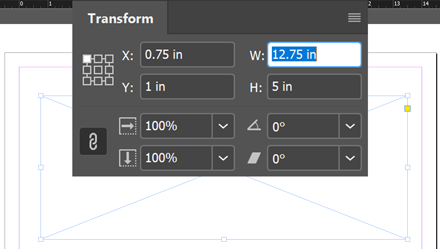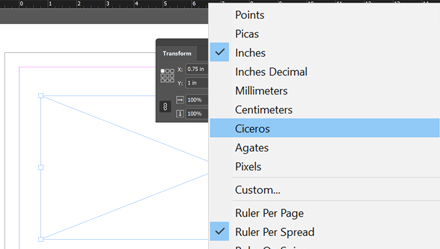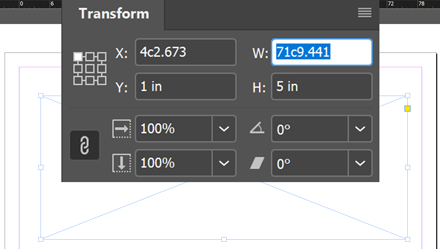## The Issue

Mikhail considered the case of setting a frame width to (exactly) `12.75in`:Now, right-clicking the horizontal ruler and changing the measurement unit to “Ciceros”,you get the value `71c9.441` in typographic notation (which means `71+9.441/12` in decimal notation.)So, according to InDesign, `12.75in ≈ 71c9.441`. The symbol `≈` accounts for the approximation.

Note. — InDesign's display precision is 1/10,000 up to 4 decimal points, and all measurements are internally coerced into PostScript points. See “Precision of HurryCover Measurements” for a more detailed discussion on this topic.

However, Mikhail's original ExtendScript code produced a slightly different result (`71c9.5866`), which deviates from the displayed value by `+0c0.1456`, that is, `+0.0121 ci` in decimal notation. This really is a tiny difference—something like `55 µm`—but Mikhail wanted to explain the gap and improve his script. Most InDesign users aren't familiar with rounding errors, so they may visually consider `71c9.5866` as a wrong value compared to `71c9.441`. In fact, they differ only by the size of three neurons!

In the previous version of the SizeInUnitsEng.jsx script, the general strategy was to apply an Adobe-to-French-Points conversion when dealing with ciceros. For that purpose, the factor `3528/3759`, or similarly `254/271` was used, based on the fact that “French inch has size 27.1 mm [resp. French point is 0.3759 mm]; while Adobe inch is 25.4 mm long [resp. Abobe point is 0.3528 mm].”

Also, it was assumed that `UnitValue`'s metric accurately reflects those ratios… which didn't appear to be the case at all!

## So, What Is a Cicero?

• According to wikipedia FR, one cicero is 12 Didot points, that is:

1 ci ≈ 4.512 mm.

(The `≈` symbol is very important here, as the definition of the “point” itself varied across History, as well as the “French inch”.)

• According to wikipedia EN, a better estimation of the cicero (“1/6 of the historical French inch”) would be:

1 ci ≈ 4.51165812456 mm.

• According to InDesign's display, 1000 ciceros equals 4511.278 mm, so:

1 ci ≈ 4.511278 mm.

• Finally, according to the `UnitValue` class implemented in InDesign/ExtendScript,

1 ci ≈ 4.51104 mm,

so we clearly get an uncertainty from the 4th decimal place when dealing with millimeters.

Regarding inches, it's worth mentioning that InDesign display is altered by rounding errors as well, so it may appear that it doesn't even comply with its own rules in some cases. Just consider the `12.75in` example above:

12.75 in ≈ 71c9.441  (ID display.)

Now, in Adobe metrics, we have two known facts:

(a) 1 in = 72pt (Adobe measurements.)

(b) 1 ci = 12.7872pt (straight from `UnitValue`.)

From (a), `12.75in = 918pt`;
from (b), `10000ci = 127872pt`,
which leads to the following conversion table:It comes `12.75 inches = (71 + 117/148) ciceros`. The decimal part expressed in 12th gives `351/37` (≈ 9.486486486), so the best approximation we can write is:

12.75 in ≈ 71c9.4865 (our result — vs. 71c9.441 from ID.)

So, according to our own calculations, InDesign's display is “wrong” by about `+0c0.04`. Still a microscopic error!

As a matter of fact, we don't know with a sufficient accuracy the internal factor that `UnitValue` uses when it converts from ciceros. However, since it is considered that 1 cicero should equal “12 French points”, the best offer we can provide from the `UnitValue` perspective is:

`const K = 12/(UnitValue("1ci").as("pt")); // 0.93843843843844`

The value of K falls between 254/271 (≈0.937269373) and 3528/3759 (≈0.938547486) mentioned earlier. There are good reasons to suppose it will bring more consistent results.

Here is a sample script that you can use for testing this approach:

```
// Proposed factor when using intermediate
// conversion to points (French vs. Adobe.)
// ---
const K = 12/(UnitValue("1ci").as("pt"));

// Input parameters
// ---
var inputString = "12.75";
var inputUnit = "in";

// Rounding param.
// ---
var round = 4;

// Conversion.
// ---
var sizeAsAbobePt = UnitValue(inputString, inputUnit).as("pt");
var sizeAsFrenchPt = sizeAsAbobePt*K;

floatResult = parseFloat(sizeAsFrenchPt/12);
intResult   = parseInt(sizeAsFrenchPt/12);

// Refine the frac. part (typographic notation.)
// ---
diff = floatResult - intResult;
frac12 = (diff*12).toFixed(round);

// Output.
// ---
alert( intResult + "c" + frac12 );  // 71c9.4865
```

This leads to a very decent approximation (`71c9.4865`) although InDesign still displays different digits (`71c9.441`) that anyway diverge from the theoretical result.

Note. — Don't be surprised if you observe differences at the 2nd or 3rd position after the decimal point in a fraction that itself represents how many 12th of the cicero unit we should take! The disagreement between `71c9.49` and `71c9.44` is, in fact, anecdotal. Since K is uncertain from the third decimal place, `10*K` is uncertain from the 2nd decimal place, `100*K` is uncertain from the 1st decimal place, and so on. In our particular example, sizeAsAbobePt is about 918 (in pt), which may even generate a bigger error. If we had used `K=254/271`, the final result would have been `71c8.4133`! And I do not mention the additional rounding errors introduced by floating-point arithmetic (multiplications and especially divisions.)

## A More Direct Solution

But, to me, there was still a mystery in Mikhail's algorithm: why on earth would the Adobe-to-French points translation have to be processed?

Indeed, is there anything wrong in just invoking `UnitValue(input).as("ci")`?

Here is the simple code I've tested while investigating the issue:

```var v = UnitValue("12.75in").as("ci");
// => 71.7905405405405 ; exact: 71 + 117/148.

// Convert decimal notation into typographic notation `#c#.#`
// ---
var n = parseInt(v,10); // Integer part: 71

var d12 = 12*(v-n);     // Dec. part × 12
// => 9.4864864864864 (exact: 351/37.)

12 <= d12 && (++n, d12-=12); // Who knows?
var result = n + 'c' + d12;

Without a shadow of a doubt, this is the closest value to the theoretical result (`71c9.4865`) ever obtained. The reason is, almost all intermediate multiplications and divisions have been avoided, the core computation being just performed by `UnitValue`'s internal processor.